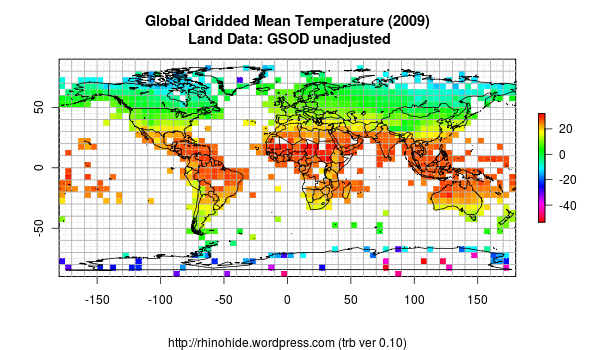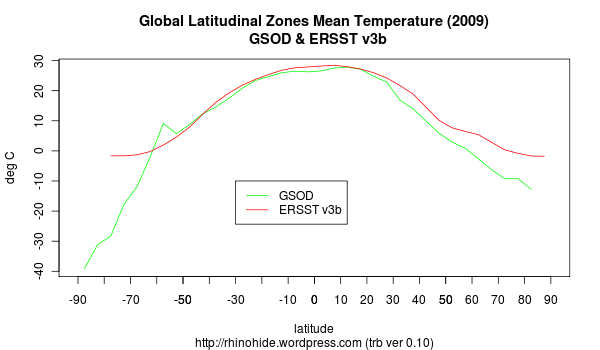Home > ERSST, GHCN, GSOD, trb > trb-0.10: GSOD debut

## trb-0.10: GSOD debut

2010 July 26

Introduction

Fairly minor changes, but I check out the GSOD data set, as well as adding some more raster plotting pieces.

Notes

I can see a point coming fairly quickly where I break this code into a set of components that read different data sorts and writes them out as raster files. And another that reads different raster files and performs various manipulations with the data.

The GHCN formatted GSOD data integrated smoothly.

Code

```###############################################################################
# https://rhinohide.wordpress.com
###############################################################################
# trb 0.10 : 2010 07 25 : Cleanup / Subroutines / Additional Displays / GSOD
# trb 0.09 : 2010 07 24 : Planet Ocean
# trb 0.08 : 2010 07 23 : Raster / Pretty Pictures / Fractional Area Weights
# trb 0.07 : 2010 07 18 : Performance Refactor (22 seconds with data read)
# trb 0.06 : 2010 07 17 : Performance Refactor (3x faster, still 8hrs for all)
# trb 0.05 : 2010 07 14 : Gridding by Latitude and Longitude
# trb 0.04 : 2010 07 13 : Proportional Latitudinal Bands
# trb 0.03 : 2010 07 12 : Weighted Latitudinal Bands
# trb 0.02 : 2010 07 11 : Simple Latitudinal Bands
# trb 0.01 : 2010 07 10 : GHCN Simple Mean Average
###############################################################################
ver <- "0.10"

# process data fresh or reuse last run
read <- FALSE # set to FALSE if this is your first time through
#read <- TRUE # set to TRUE if you have previous data to read

# land setup
flag_land <- FALSE # set to FALSE to exclude land data
flag_land <- TRUE # set to TRUE to include land data
land_data <- "../data/my.gsod.mean" # assumes a GHCN style v2.mean file
land_inv <- "../data/my.gsod.inv" # assumes a GHCN style inv file

# ocean setup
flag_ocean <- FALSE # set to FALSE to exclude ocean data
flag_ocean <- TRUE # set to TRUE to include ocean data
ocean_data <- "../data/my.ersst.mean.all" # assumes a GSOD style v2.mean file
ocean_inv <-  "../data/my.ersst.inv" # assumes a GSOD style inv file

# grid geometry
grid_bands <- 36 # number of latitudinal bands
grid_bars <- 72 # number of longitudinal bars
#flag_latarea <- TRUE # set to TRUE if you want equal area lat bands
flag_latarea <- FALSE # set to FALSE if you want equal step lat bands

###############################################################################
# Read Data Files
###############################################################################
# ftp://ftp.ncdc.noaa.gov/pub/data/GSOD/v2/v2.mean
# ftp://ftp.ncdc.noaa.gov/pub/data/GSOD/v2/v2.temperature.inv
# ??? url for ersst
###############################################################################

# this flag is to indicate if we must read for the first time
# or if we can read the data from previous runs

# TO-DO split into land read and ocean read ?

if (flag_land) {

#----------------------------------------------------------------------------
# Land Temperature File (GSOD formatted monthly means)
#----------------------------------------------------------------------------

# mm for 'monthly means'
lmmwids=c(11,1,4,5,5,5,5,5,5,5,5,5,5,5,5)
lmmcols=c("id","dupe","year","jan","feb","mar","apr","may","jun",
"jul","aug","sep","oct","nov","dec")
# read the file
lmm <- read.fwf(land_data, widths=lmmwids, col.names=lmmcols,

#----------------------------------------------------------------------------
# Inventory File
#----------------------------------------------------------------------------

# there are five occurrances of the pound hash in v2.temperature.inv
# R scripts chokes on reading those, remove them first

linvcols <-c("id","name","lat","lon","alt","elev","ru","pop","terr","arid",
linvwids <- c(11,32,6,8,5,5,1,5,2,2,2,2,1,2,16,1,5)

} # end read land

if (flag_ocean) {
#----------------------------------------------------------------------------
# Ocean Temperature File (GSOD formatted monthly means)
#----------------------------------------------------------------------------
# I'm cheating a little here, will need to handle import of native format
# but for now, I am using the ERSST data I formatted as GSOD earlier

# mm for 'monthly means'
ommwids=c(11,1,4,5,5,5,5,5,5,5,5,5,5,5,5)
ommcols=c("id","dupe","year","jan","feb","mar","apr","may","jun",
"jul","aug","sep","oct","nov","dec")

# read the file

#----------------------------------------------------------------------------
# Inventory File
#----------------------------------------------------------------------------

oinvcols <-c("id","name","lat","lon","alt","elev","ru","pop","terr","arid",
oinvwids <- c(11,32,6,8,5,5,1,5,2,2,2,2,1,2,16,1,5)

} # end read ocean

###############################################################################
# Set up arrays for latitudinal banding
###############################################################################

# takes GSOD station inventory and list of stations
# returns lat, lon array of station coordinates
find_inv_coordinates <- function (stninv, stndata) {

# build a hash table
hlat=new.env(hash=TRUE)
hlon=new.env(hash=TRUE)
for (i in 1:nrow(stninv)) {
hlat[[as.character(stninv[i,1])]] <- stninv[i,3]
hlon[[as.character(stninv[i,1])]] <- stninv[i,4]
}

# array for station lat lon
stnll <- matrix(NA,length(stndata),2)

# assign lats and lons from hash table
for (i in 1:length(stndata)) {
stnll[i,1] <- hlat[[as.character(stndata[i])]]
stnll[i,2] <- hlon[[as.character(stndata[i])]]
}

hlat <- NULL
hlon <- NULL

return (stnll)
}

# bind lat lon coordinates to data file
if (flag_land) { lmm1 <- cbind(lmm,find_inv_coordinates(linv,lmm[,1])) }
if (flag_ocean) { omm1 <- cbind(omm,find_inv_coordinates(oinv,omm[,1])) }

nrow(lmm1) # debug

# ---------------------------------
# grid geometry
# ---------------------------------
# general formula for a set number of latitude bands of equal area
bands <- rep(NA,grid_bands)
b = 90                      # start with santa
bands=b
bands[grid_bands+1]=-90         # rounding errors at grid_bands ~ 32
for (i in 1:(grid_bands-1)) {
if (flag_latarea) {
# equal area lat bands
a = asin(sin(b*pi/180)-2/grid_bands)
b = a * 180 / pi
bands[i+1] = b
} else {
# equal lat step size
bands[i+1] = 90 - (180/grid_bands) * i
}
}
bands

# 'bars' are the longitudinal edges of the grid
# calculate the bars long now so we can just lookup later
bars <- matrix(NA,grid_bands+1,grid_bars+1)
bars[,1] = -180            # start in Howland Island and move east ;-)
for (i in 1:(grid_bands+1)) {
for (j in 1:grid_bars) {
bars[i,j+1] = 360*(j/grid_bars)-180
}
}
bars[1,]

## weights for each cell
weights <- matrix(NA,grid_bands,grid_bars)
for (i in 1:(grid_bands)) {
for (j in 1:grid_bars) {
# assumes equal cells within a lat ban
weights[i,j]<-abs((sin(bands[i]*pi/180)-sin(bands[i+1]*pi/180)))/(2*grid_bars)
}
}
sum(weights[,]) # should equal 1

#------------------------------------------------------------------------------
# assign each record to a grid point
#------------------------------------------------------------------------------
# lat, lon, year, stn annual mean

fm1 <- function(i) { sum(bands>i) }
# sensitive to end points (-180,180)
#fm2 <- function(j) { floor( (j + 180) * grid_bars / 360 ) +1 }
fm2 <- function(j) { ceiling( (j + 180) * grid_bars / 360 ) }

get_cell_coordinates <- function(l1,l2) {
ill <- matrix(NA,length(l1), 2)
ill[,1] <- as.integer(lapply(l1,fm1))
ill[,2] <- as.integer(lapply(l2,fm2))
return(ill)
}

if (flag_land) {
# temps are stored as 10ths C
lmm2 <- cbind(get_cell_coordinates(lmm1[,16],lmm1[,17]),
lmm1[,3], rowMeans(lmm1[,4:15],na.rm=T)/10)
colnames(lmm2) <- c("ilat","ilon","yr","mean")
}

if (flag_ocean) {
# temps are stored as 100ths C
omm2 <- cbind(get_cell_coordinates(omm1[,16],omm1[,17]),
omm1[,3], rowMeans(omm1[,4:15],na.rm=T)/100)
colnames(omm2) <- c("ilat","ilon","yr","mean")
}

#------------------------------------------------------------------------------
# set up the range of years to calculate
#------------------------------------------------------------------------------
if ( flag_ocean && flag_land ) {
# find common overlap
year0 <- max(min(lmm1[,3]),min(omm1[,3]))
year1 <- min(max(lmm1[,3]),max(omm1[,3]))
} else if (flag_land) {
year0 <- min(lmm1[,3])
year1 <- max(lmm1[,3])
} else if (flag_ocean) {
year0 <- min(omm1[,3])
year1 <- max(omm1[,3])
}

# or override the above
#year0 <- 1880
#year1 <- 2009

# calculate the range of years
range <- year1 - year0 + 1

#------------------------------------------------------------------------------
# trim the matrix to the span of years
#------------------------------------------------------------------------------
trim_matrix <- function(mat, y0, y1) {
idx <- mat[,3]>= y0 & mat[,3]<=y1
return(mat[idx,])
}

if (flag_land) { lmm2 <- trim_matrix(lmm2, year0, year1) }
if (flag_ocean) { omm2 <- trim_matrix(omm2, year0, year1) }

#------------------------------------------------------------------------------
# clean up
#------------------------------------------------------------------------------
hlat <- NULL
hlon <- NULL

if (flag_land) {
lmm <- NULL
lmm1 <- NULL
linv <- NULL
}

if (flag_ocean) {
omm <- NULL
omm1 <- NULL
oinv <- NULL
}

#------------------------------------------------------------------------------
# write the data
#------------------------------------------------------------------------------

if (flag_land) { write.table(lmm2,".Rlmm2",row.names=F,col.names=T) }
if (flag_ocean) { write.table(omm2,".Romm2",row.names=F,col.names=T) }
write.table(bands,".Rbands",row.names=F,col.names=F)
write.table(bars,".Rbars",row.names=F,col.names=F)
write.table(weights,".Rweights",row.names=F,col.names=F)
write.table(c(year0,year1),".Ryears",row.names=F,col.names=F)

} else {

#------------------------------------------------------------------------------
# read the data
#------------------------------------------------------------------------------

if (flag_land) { lmm2 <- read.table(".Rlmm2",header=T) }
if (flag_ocean) { omm2 <- read.table(".Romm2",header=T) }

grid_bands <- length(bands) -1
grid_bars <- ncol(bars) -1
year0 <- yrs
year1 <- yrs
range <- year1 - year0 + 1

} # end if for data reads

# this is a trick to ensure that some value (NA) exists for every lat band
# required to ensure that the tapply mean factors every lat band
a1 <- matrix(NA,grid_bands,4)
a1[,1] <- rep(1:grid_bands)
a1[,2] <- 1
a1[,3] <- year0
colnames(a1) <- c("ilat", "ilon", "yr", "mean")
if (flag_land) { lmm2 <- rbind(lmm2,a1) }
if (flag_ocean) { omm2 <- rbind(omm2,a1) }
a1 <- NULL

###############################################################################
# Calculate Annual Mean Average
###############################################################################

# convenient list of years for later use
lyear <- seq(year0:year1) + year0 -1

#------------------------------------------------------------------------------
# grid the temperature data
#------------------------------------------------------------------------------
if (flag_land) {
lgmean <- array(NA, dim=c(grid_bands,grid_bars,range)) # holds the mean of each cell
lgmean <- tapply(lmm2[,4], list(lmm2[,1],lmm2[,2],lmm2[,3]), mean, na.rm=T)
}

if (flag_ocean) {
ogmean <- array(NA, dim=c(grid_bands,grid_bars,range))  # holds the mean of each cell
ogmean <- tapply(omm2[,4], list(omm2[,1],omm2[,2],omm2[,3]), mean, na.rm=T)
}

###############################################################################
# Raster Display
###############################################################################

library(sp)
library(rgdal)
library(raster)
library(maps)

# take 2 rasters and return the mean
mean_raster_values <- function(r1,r2) {
# TO-DO error check to ensure both are same size
rr <- matrix(nrow=nrow(r1), ncol=ncol(r1))
for (r in 1:nrow(rr)) {
rr[r,] <- colMeans(rbind(getValues(r1,r),getValues(r2,r)), na.rm=T)
}
return(rr)
}

# take 2 rasters and return the mean
plot_raster_values <- function(r1,title,subt) {
plot(r1,col=rev(rainbow(256)), main=title, sub=subt)
grid(nx=grid_bars,ny=grid_bands,col="lightgray")
grid(nx=grid_bars/2,ny=grid_bands/2,col="darkgray",lty="solid")
}

# plot latitudinal mean
plot_raster_lat_means <- function(r1,title,subt) {
rlats <- get_raster_lat_means(r1)
llats <- bands[1:grid_bands] + 90/grid_bands
plot(rlats ~ llats, main=title, sub=subt)
}

# get latitudinal means
get_raster_lat_means <- function(r1) {
rlats <- rep(NA,nrow(r1))
for (r in 1:length(rlats)) {
rlats[r] <- mean(getValues(r1,r), na.rm=T)
}
return(rlats)
}

# get global mean (with very simplistic interpolation)
get_global_lat_mean <- function(lm) {
# get first and last values not null
mask <- ! is.na(lm)
# interpolate NA polar zones
if ( is.na(lm) ) { lm <- mna }
if ( is.na(lm[grid_bands]))  { lm[grid_bands] <- mna[length(mna)] }
for (i in 2:(grid_bands-1)) {
if (is.na(lm[i])){lm[i]=(lm[i-1]+lm[i+1])/2}
}
m <- mean( lm * weights[,1]*grid_bars * grid_bands, na.rm=T)

return(m)
}

if ( flag_land ) {

lr <- raster(nrows=grid_bands,ncols=grid_bars,
xmn=bars[1,1],xmx=bars[1,grid_bars+1],
ymn=bands[grid_bands+1],ymx=bands,
crs="+proj=longlat +datum=WGS84")
# set a year to display
y <-range # last year
lr <- setValues(lr,as.vector(t(lgmean[,,y])),layer=1)
get_global_lat_mean(get_raster_lat_means(lr)) # 16.39112 # 15.11366

img=paste("trb-",ver,"-land-grid-",lyear[y],".png", sep="")
tit=paste("Global Gridded Mean Temperature (",lyear[y],
")\n Land Data: GSOD unadjusted",sep="")
subt=paste("https://rhinohide.wordpress.com (trb ver ",ver,")",sep="")
png(img, width=600, height=350)

plot_raster_values(lr,tit,subt)

}

if ( flag_ocean ) {

or <- raster(nrows=grid_bands,ncols=grid_bars,
xmn=bars[1,1],xmx=bars[1,grid_bars+1],
ymn=bands[grid_bands+1],ymx=bands,
crs="+proj=longlat +datum=WGS84")
# set a year to display
y <-range
or <- setValues(or,as.vector(t(ogmean[,,y])),layer=1)
get_global_lat_mean(get_raster_lat_means(or)) # 17.85739

img=paste("trb-",ver,"-ocean-grid-",lyear[y],".png", sep="")
tit=paste("Global Gridded Mean Temperature (",lyear[y],
")\n Ocean Data: ERSSTv3",sep="")
subt=paste("https://rhinohide.wordpress.com (trb ver ",ver,")",sep="")
png(img, width=600, height=350)

plot_raster_values(or,tit,subt)
}

# plot both land and ocean
if ( flag_land && flag_ocean ) {

lor <- raster(nrows=grid_bands,ncols=grid_bars,
xmn=bars[1,1],xmx=bars[1,grid_bars+1],
ymn=bands[grid_bands+1],ymx=bands,
crs="+proj=longlat +datum=WGS84")
# set a year to display
y <-range
lor <- setValues(lor,as.vector(t(mean_raster_values(lr,or))),layer=1)
get_global_lat_mean(get_raster_lat_means(lor)) # 16.79416 # 16.49551

img=paste("trb-",ver,"-land-ocean-grid-",lyear[y],".png", sep="")
tit=paste("Global Gridded Mean Temperature (",lyear[y],
")\n Land Data: GSOD unadjusted / Ocean Data: ERSSTv3",sep="")
subt=paste("https://rhinohide.wordpress.com (trb ver ",ver,")",sep="")
png(img, width=600, height=350)

plot_raster_values(lor,tit,subt)
}

# plot land and ocean overlap
if ( flag_land && flag_ocean ) {

mask1 <- ! is.na(getValues(lr))
mask2 <- ! is.na(getValues(or))
aav <- as.vector(getValues(lor)) * mask
lor2 <- raster(nrows=grid_bands,ncols=grid_bars,
xmn=bars[1,1],xmx=bars[1,grid_bars+1],
ymn=bands[grid_bands+1],ymx=bands,
crs="+proj=longlat +datum=WGS84")
lor2 <- setValues(lor,aav,layer=1)
img=paste("trb-",ver,"-land-ocean-grid-overlap-",lyear[y],".png", sep="")
tit=paste("Global Gridded Mean Temperature Overlap (",lyear[y],
")\n Land Data: GSOD unadjusted / Ocean Data: ERSSTv3b",sep="")
subt=paste("https://rhinohide.wordpress.com (trb ver ",ver,")",sep="")
png(img, width=600, height=350)

plot_raster_values(lor2,tit,subt)

}

# plot land and ocean latitudinal zones
if ( flag_land && flag_ocean ) {

img=paste("trb-",ver,"-lat-zones-",lyear[y],".png", sep="")
tit=paste("Global Latitudinal Zones Mean Temperature (",lyear[y],
")\n  GSOD & ERSST v3b",sep="")
subt=paste("https://rhinohide.wordpress.com (trb ver ",ver,")",sep="")
png(img, width=600, height=350)

rlats1 <- get_raster_lat_means(lr)
rlats2 <- get_raster_lat_means(or)
llats <- bands[1:grid_bands] - 90/grid_bands
plot(rlats1 ~ llats, type="l", col="green", main=tit, sub=subt,
xlim=c(-90,90), ylab="deg C", xlab="latitude")
lines(rlats2 ~ llats, col="red")
axis(1, seq(-90,90,10))
legend( -30,-30, c("GSOD","ERSST v3b"), lty=c(1,1), col=c("green","red"))

}
```

trb-0.10.R

Results1. 2010 July 26 at 5:47 am

You psychopath, you just drowned all of Scandinavia in cold water. What did they ever do to you?

Anyway, i like the rainbow bar.

2. 2010 July 26 at 6:12 am

What can I say? My Swedish forefathers sold out. 😉

Ever hear of ‘bandy?’
http://www.brobergsoderhamn.se/

3. 2010 August 2 at 11:38 am

Two questions:

1) When are you going to write something up and submit it to a peer-reviewed journal??? This is a really nice contribution to the whole surface temperature record debate, and it would be nice to have it available for citation as a counter-argument to the “but HadCRUT, NASA, and NOAA all use GHCN!” argument for those of us who aren’t allowed to use blogosphere science (even though our opponents have no such restrictions).

2) How many stations are in both GHCN (either through CLIMAT or the Peterson/Vose 1992 effort) and GSOD? And if there is overlap, can you do an analysis only with those stations which are _not_ in GHCN?

Thanks!

-M

4. 2010 August 4 at 7:23 am

Rainbow. love it

1. No trackbacks yet.